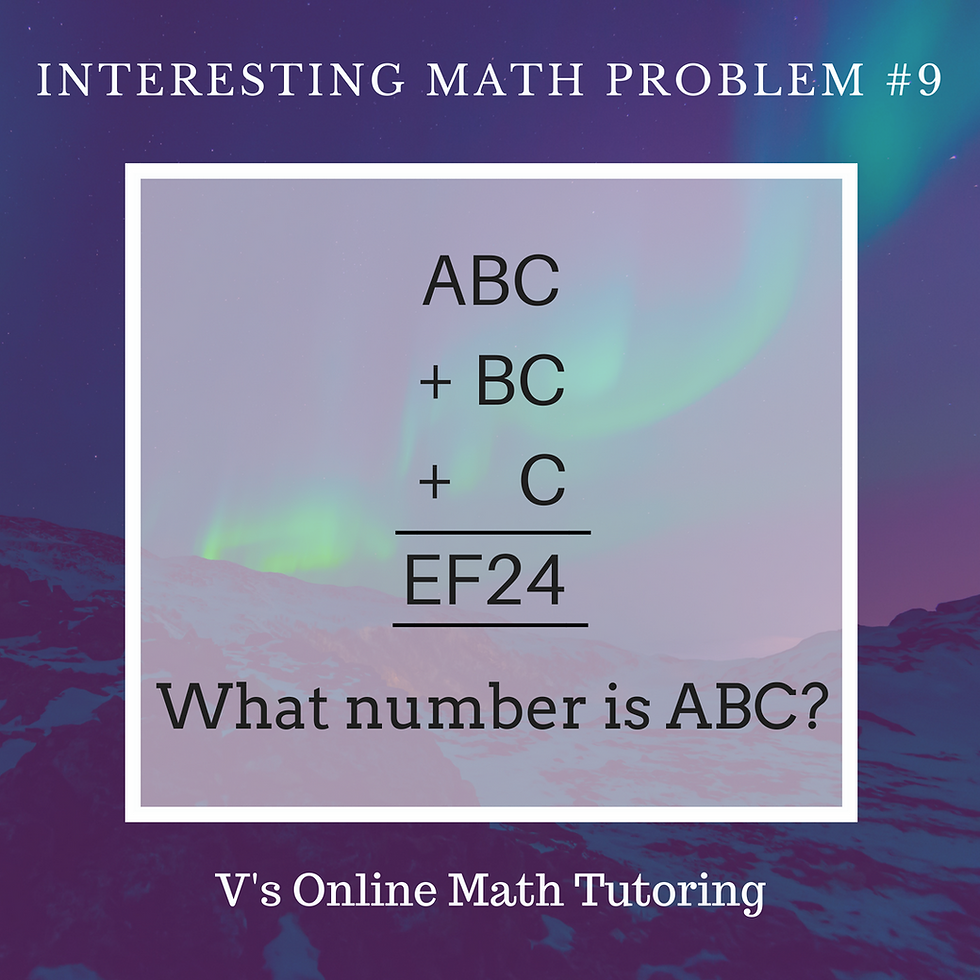top of pageSearch

# Math Competition Problems Problem of the Week - 8

Updated: May 11, 2021

2BA + C6D  = 8AD. Find out A, B, C and D

Source: Adapted from CML math competitionMath Competition Problems - Problem of the Week 8

## Solution:

First let's see the unit's place. For A+D=D, A has to be 0 (zero). This is the identity property of addition. Any number plus zero gives itself. No other number other than zero has this property. Hence A=0 and D can be any number.

Next B+6=A and A=0. So B+6=0. Since B is a positive number, B+6 = 10. So there should be a carry over. And 4+6=10, hence B=4

Now 2+C+1 (carry over) = 8.

So, 3+C=8. Hence C=5Interesting Math Problems

Like what you see? Would you like to have the Problem of the Week delivered to your inbox? Click here to subscribe

As you know I'm an Online Math Tutor. I coach kids for all elementary and middle school math competitions. This is a problem my average 3rd or 4th grader would be able to solve. To know more about me and how I work click here.